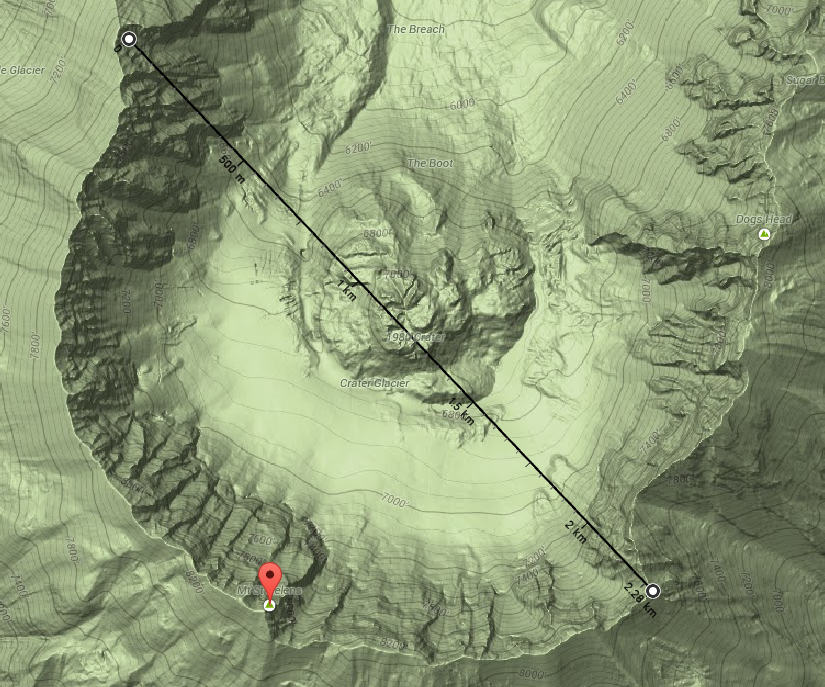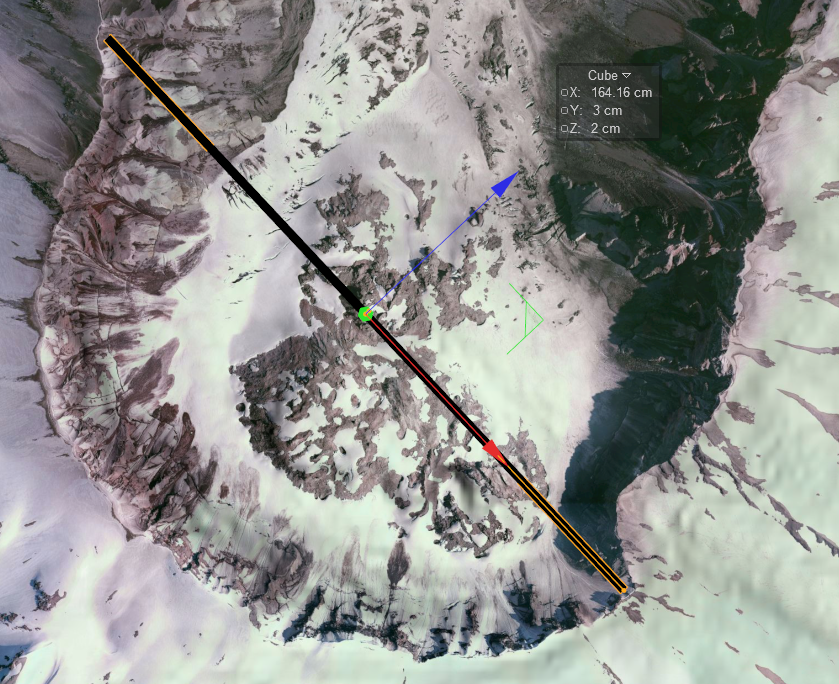To place an object, of an exact size onto DEM Earth, use the World Info/Object.Unit.Meter as a multiplier.

For example, you want to place an object onto DEM earth, that is exactly 10M in real world length,width and height.

make a cube, set its c4d size to 1000 meters  (make sure your units are meters,etc)

lets say the DEM earth object has an object.unit.scale of 0.072 , you would then multiply 1000 by 0.072, which is 72.

so you set the size of you cube to 72, 72,72 and place the object on the DEM Earth surface.

you then need to place this cube under a pinpoint, to lock it to the surface.

example:

google maps says that the following distance is 2.28 kmDEM Earths unit scale is 0.072

So we set the length of this cube to 2280 * 0.072 , which is 64.16m

And we can see that the cube is now pretty much exactly the right length.